# Martina

Martina is solving the equation 4x - 11 = 2x + 391. Here are the first steps of her solution.

4x - 11 = 2x + 391

2x - 11 = 391

2x = 402

What did Martina do to get 2x - 11 = 391?

x =  201

### Step-by-step explanation: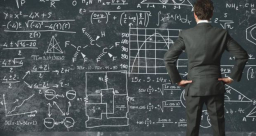Did you find an error or inaccuracy? Feel free to write us. Thank you!

Showing 1 comment:Marie
Answer to previous question is: She subtracted 2x from both sides of equation. 4x-2x=2x on left and 2x-2x = 0 on right.Tips to related online calculators
Do you have a linear equation or system of equations and looking for its solution? Or do you have a quadratic equation?

## Related math problems and questions:

• RemaindersIt is given a set of numbers { 170; 244; 299; 333; 351; 391; 423; 644 }. Divide this numbers by number 66 and determine set of remainders. As result write sum of this remainders.
• Emily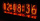Emily had 20 minutes to do a three-problem quiz. She spent 11 3/4 minutes on question A and 5 1/2 minutes on question B. How much time did she have left for question C?
• ArelliArelli had 20 minutes to do a three-problem quiz. She spent 9 7/10 minutes on question A and 3 2/5 minutes on question B. How much time did she have left for question C? Solve on paper to find the answer as a fraction.
• Francesca 2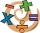Francesca applied the steps below to find the product of (-1.2)(-9.4). Step 1: (-1.2)(-9.4) = (-9.4)(-1.2) Step 2:                   = (-9.4)(-1) + (-9.4)(-0.2) Step 3:                   = (9.4) + (1.88) Step 4:                   = 11.28 Which step shows
• Logik game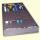Letter game Logik is a two player game, which has the following rules: 1. The first player thinks five-letter word in which no letter is not repeated. 2. The second player writes a five-letter word. 3. The first player answers two numbers - the first numb
• Sphere from tree pointsEquation of sphere with three point (a,0,0), (0, a,0), (0,0, a) and center lies on plane x+y+z=a
• Four prismsQuestion No. 1: The prism has the dimensions a = 2.5 cm, b = 100 mm, c = 12 cm. What is its volume? a) 3000 cm2 b) 300 cm2 c) 3000 cm3 d) 300 cm3 Question No.2: The prism base is a rhombus with a side length of 30 cm and a height of 27 cm. The height of t
• The petAnanya has a bunny. She bought 4 7/8 pounds of carrots. She fed her bunny 1 1/4 pounds of carrots the first week. She fed her bunny 5/6 pounds of carrots the second week. All together, how many pounds of carrots did she feed her bunny? 1. Draw a tape diag
• Fraction to decimalWrite the fraction 3/22 as a decimal.
• Honey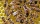Ila collected the honey from 3 of her beehives. From the first hive, she collected 2/3 gallons of honey. The last two hives yielded 1/4 gallon each. After using some of the honey she collected for baking, Lila found that she only had 3/4 gallon of honey l
• Coordinates of the vertices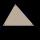Calculate the coordinates of the vertices of a triangle if the equations of its sides are 7x-4y-1 = 0 x-2y + 7 = 0 2x + y + 4 = 0
• Three digits number 2Find the number of all three-digit positive integers that can be put together from digits 1,2,3,4 and which are subject to the same time has the following conditions: on one positions is one of the numbers 1,3,4, on the place of hundreds 4 or 2.
• KrishnaKrishna bought 5 1/2 meters of ribbon from the market . She cut off a 2 3/4 m piece of ribbon from it and gave it to Sushima . What length of ribbon is left with her?
• Solve 2Solve integer equation: a +b+c =30 a, b, c = can be odd natural number from this set (1,3,5,7,9,11,13,15)
• Reminder and quotientThere are given numbers A = 135, B = 315. Find the smallest natural number R greater than 1 so that the proportions R:A, R:B are with the remainder 1.
• DigitsHow many odd four-digit numbers can we create from digits: 0, 3, 5, 6, 7? (a) the figures may be repeated (b) the digits may not be repeated
• Subtract 4Subtract the following decimals. a. 0.98 - 0.053 b. 0.67 - 0.4 c. 0.3 - 0.002 d. 3.2 - .789 e. 6.53 - 4.298 f. 6 - 4.32 g. 7 - 3.574 h. 4.83 - 1.8 i. 3.7 - 1.8 j. 16.17 - 11.632